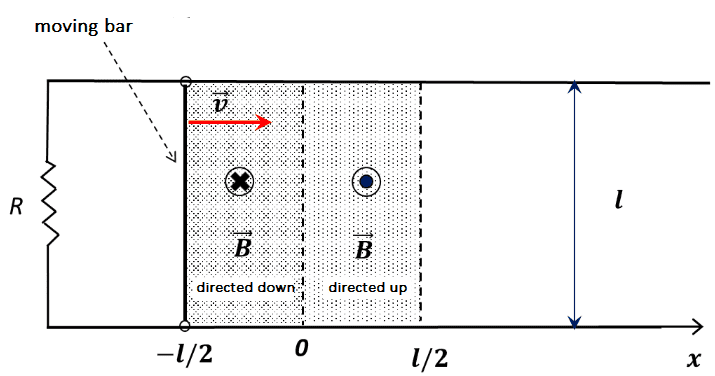# Magnetic flux with magnetic field changing direction

• besebenomo
I can't think of a conceptual justification either, but I think it might be because the flux might be smaller.The flux might be smaller.f

#### besebenomo

Homework Statement
In the circuit shown in picture, having resistance R, a bar of mass m and lenght l moves without friction with velocity v= l/T. The circuit is at time t=0 in a regione with no magnetic field, then in the first part (-l/2<= x <0) the magnetic field is constant and equal to -B0, later it changes to +B0 (0< x <=l/2). We are considering what happens during 0<t<=T. Find current flowing in the circuit, force that needs to be applied to keep the bar moving at constant speed and (something else I don't know how to traslate in english: power balance maybe?)
Relevant Equations
Sorry if I post again about this topic (last time I promise!) but I still have some doubts regarding the concept of flux. This collection of problems I have quite standard but there are so many variations. Here is the circuit in question:Something tells me that I could write a function that spans the whole region and then integrate... Maybe I could use ##B(x) = |B_{0}|##, but doesn't look convenient... So I just do as if those two regions were independent.

Region ##-\frac{l}{2} \leq x<0:## the bar moves to the right while the magnetic force opposes the movement. Not sure if it's going to get in the second region, but I guess so otherwise the problem would not be like this. The flux is : ##\Phi = -B_{0}lx(t)##, the current is computed with Faraday's law and it flows in clock direction.

##x = 0:## Here the flux should be discontinous... Does it really matter?

Region ##0 \leq x<\frac{l}{2}:## supposing the bar comes in this region with a positive velocity, then the current should flow in anticlock direction. Given the fact that the initial velocity is given as ##\frac{l}{T}## and the whole thing happens in a time between 0 and T, I think that at some point the bar will stop. But these were just my thoughts, this problem looks a bit long to solve but not impossible, I am going to solve it afterwards...

##\textbf{My doubt actually is:}## if I change the ##x = 0## and move it to ##-l/2##, can I write the flux as only one function? I mean ##\Phi = B_{0}lx(t)## with ##0<=x<l/2, l/2 < x <= l## or is it more complex than that and I am missing something?

The magnetic field is clearly discontinuous. What else is discontinuous as a result? As with the other two similar problems that you posted, find separate expressions for the acceleration in the two regions using Newton's second law. If the acceleration is discontinuous at ##x=0##, then you will have to treat the motion in the two regions separately with the bar receiving a "jerk" in one direction or another at the boundary. If the acceleration is continuous, then the motion will be smooth across the boundary.

•besebenomo
The magnetic field is clearly discontinuous. What else is discontinuous as a result?
Flux, and then the derivative of the flux doesn't exist at that point, so no e.m.f. But, before doing any computations, I wanted to understand it more conceptually.

The bar in the first region should decelerate... Then there is no force in 0, and if it didn't stop before reaching that point, then the new force that acts on it is again against the movement, so the only thing that changes should be the direction of flow of the current. If I understood correctly...

However, does the flux from the first region and the second region add up?
with the bar receiving a "jerk" in one direction or another at the boundary
What do you mean? I don't think I have ever studied anything like this

Conceptually you're halfway there. You say that when the bar crosses x = 0, there is a "new force" acting on it. How does that force differ from the "old force"? You say that it is again opposing the motion, so there is no difference in direction and I agree. What about the magnitude of the new force? Is it the same or different?

A "jerk" is an informal name given to the derivative of the acceleration. It is commonly used but not found in textbooks.

•besebenomo
Thank you again for the reply
How does that force differ from the "old force"?
Force is ##\vec{F} = i \vec{l} \times \vec{B}##, the only thing that changed in here is the current which is the derivative wrt time of the (total?) flux divided by R.

I just can't make my mind whether in region 2, ##\Phi(B)_{region1}## and ##\Phi(B)_{region2}## can be added up or cancel each others out, but the latter doesn't make sense otherwise there would be no e.m.f. and no current and no force in region 2. Is it correct that they just add up?

You didn't answer my question. Forget the flux. The bar doesn't give a hoot about it. All it knows and responds to is the force acting on it. How does the force acting on the bar change from one region to the other?

•besebenomo
You didn't answer my question. Forget the flux. The bar doesn't give a hoot about it. All it knows and responds to is the force acting on it. How does the force acting on the bar change from one region to the other?
Force might be smaller?

Force might be smaller?
Why not larger? What is your justification? If you can't think of a conceptual justification, find an expression for the force in the two regions and compare.